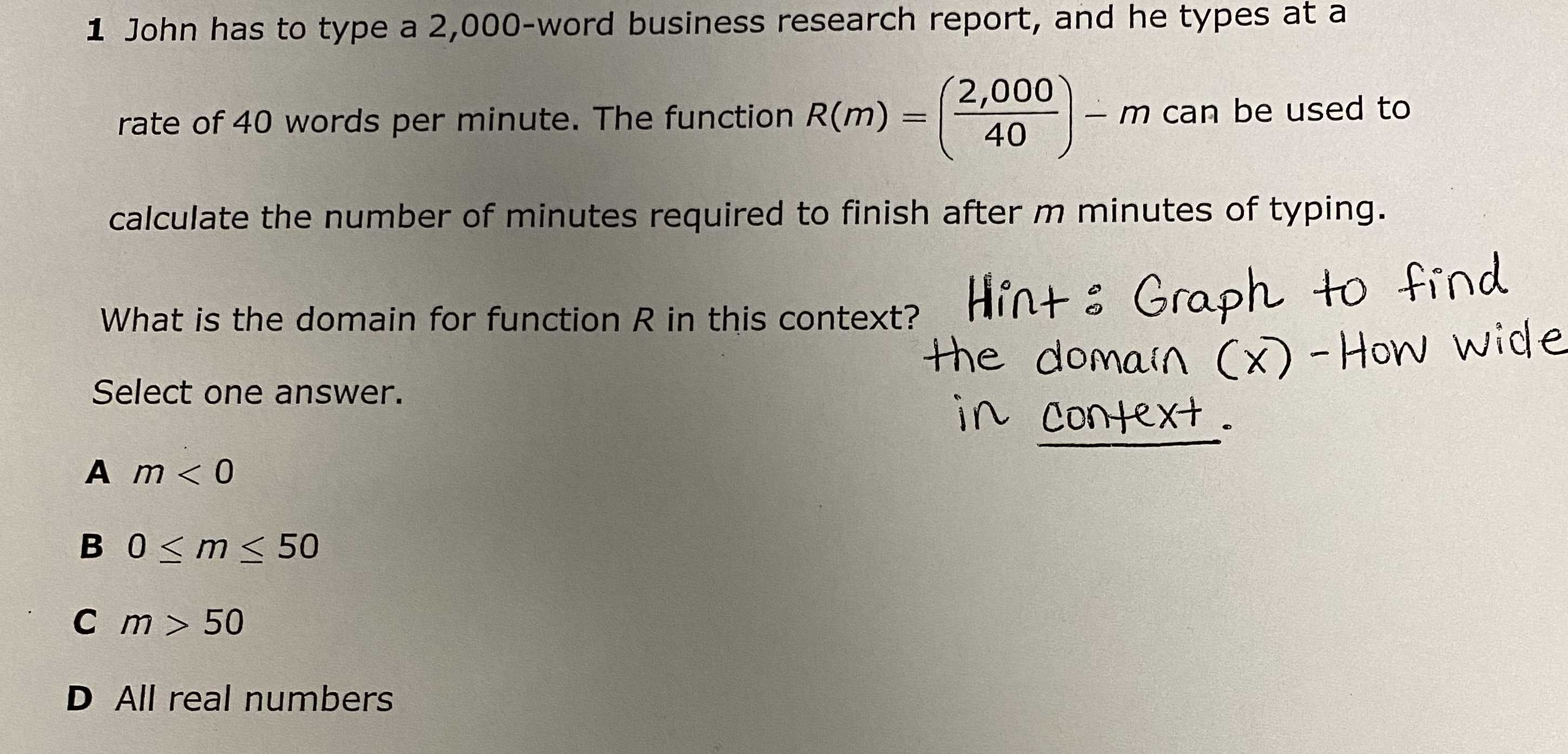### ¿Todavía tienes preguntas de matemáticas?

Pregunte a nuestros tutores expertos
Algebra
Pregunta$$1$$ John has to type a $$2,000$$ -word business research report, and he types at a rate of $$40$$ words per minute. The function $$R ( m ) = ( \frac { 2,000 } { 40 } ) - m$$ can be used to calculate the number of minutes required to finish after $$m$$ minutes of typing. What is the domain for function $$R$$ in this context? Hint: Graph to find Select one answer. ine domain $$( x ) -$$ How wide

A $$m < 0$$

$$0 \leq m \leq 50$$

$$m > 50$$

D All real numbers